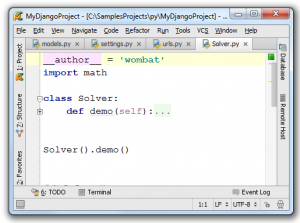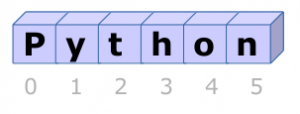# Category: Beginner

## Introduction

Welcome to my Python Course!

Python is a general-purpose computer programming language.
This course is suitable for both Python 2 and Python 3.

Related Course:
Python Programming Bootcamp: Go from zero to hero

To run Python code, you will need one of these programs:

For terminal only: Apple Mac OS X, Microsoft Windows, Linux/UNIX

<caption id=”attachment_3478” align=”alignnone” width=”300”]PyCharm, a popular Python editor

## Run Python code

A python program should be save as a file with a .py extension.
Try this code:

Expected output:

If you are using the interpreter use:

## Python stringsa string, series of characters

A string is a series of characters, they are mostly used to display text.

To define a string simply type text between quotes. Python accepts single, double and triple quotes.

Related Course:
Python Programming Bootcamp: Go from zero to hero

## String input and output

To output text (string) to the screen:

To get text from keyboard:

If you use an old Python version (2.x), you need to use:

python –version

## String Comparison

To test if two strings are equal use the equality operator (==).

To test if two strings are not equal use the inequality operator (!=)

If you are new to Python programming, I highly recommend this book.

## String Slices (part 2)

A string is a series of characters, they are mostly used to display text.

To define a string simply type text between quotes. Python accepts single, double and triple quotes.

Related Course:
Python Programming Bootcamp: Go from zero to hero

## String Index

Python indexes the characters of a string, every index is associated with a unique character. For instance, the characters in the string ‘python’ have indices:

## Characters in string

The 0th index is used for the first character of a string. Try the following:

## String Slicing

Given a string s, the syntax for a slice is:

The startIndex is the start index of the string. pastIndex is one past the end of the slice.

If you omit the first index, the slice will start from the beginning. If you omit the last index, the slice will go to the end of the string. For instance:

## Python variables

Variables can hold numbers that you can use one or more times.

Numbers can be of one of these datatypes:

• integer (1,2,3,4)

• float (numbers behind the dot)

• boolean (True or False)

Related Course:
Python Programming Bootcamp: Go from zero to hero

## Numeric variables example

Example of numeric variables:

You can output them to the screen using the print() function.

Python supports arithmetic operations like addition (+), multiplication (*), division (/) and subtractions (-).

More mathematical operations

## User input

Python 3
Use the input() function to get text input, convert to a number using int() or float().

Python 2 (old version)
You can also ask the user for input using the raw_input function:

If you are new to Python programming, I highly recommend this book.

## Python lists

Lists is a sequence and a basic data structure.   A list may contain strings (text) and numbers.  A list is similar to an array in other programming languages, but has additional functionality.

Related Course:
Python Programming Bootcamp: Go from zero to hero

## Python List

We define lists with brackets []. To access the data, these same brackets are used.
Example list usage:

We can use the functions append() and remove() to manipulate the list.

## Sort list

We can sort the list using the sort() function.

If you want to have the list in descending order, simply use the reverse() function.

If you are new to Python programming, I highly recommend this book.

## If statements

In Python you can define conditional statements, known as if-statements.
A block of code is executed if certain conditions are met.

Related Course:
Python Programming Bootcamp: Go from zero to hero

## If statements

Consider this application, it executes either the first or second code depending on the value of x.

If you set x to be larger than 10, it will execute the second code block.   We use indentation (4 spaces) to define the blocks.

A little game:
A variable may not always be defined by the user, consider this little game:

## Conditional operators

A word on conditional operators

Do not confuse the assignment operator (=) with the equals operator (==).

## Nesting

The most straightforward way to do multiple conditions is nesting:

 Operator Description != not equal == equals > greater than < smaller than
 `a = 12b = 33if a > 10: if b > 20: print("Good")`

This can quickly become difficult to read, consider combining 4 or 6 conditions.  Luckily Python has a solution for this, we can combine conditions using the and keyword.

Sometimes you may want to use the or operator.

If you are new to Python programming, I highly recommend this book.